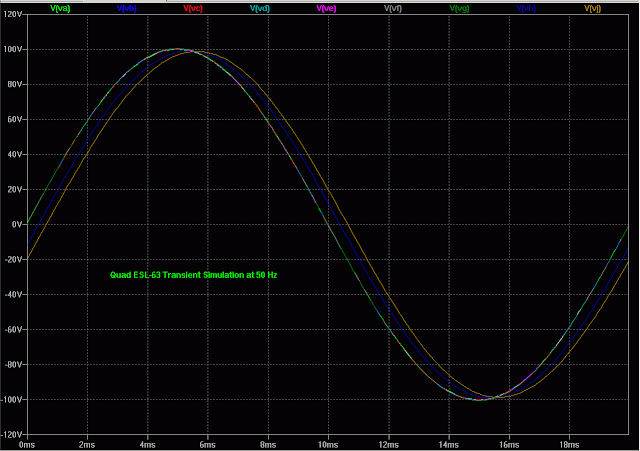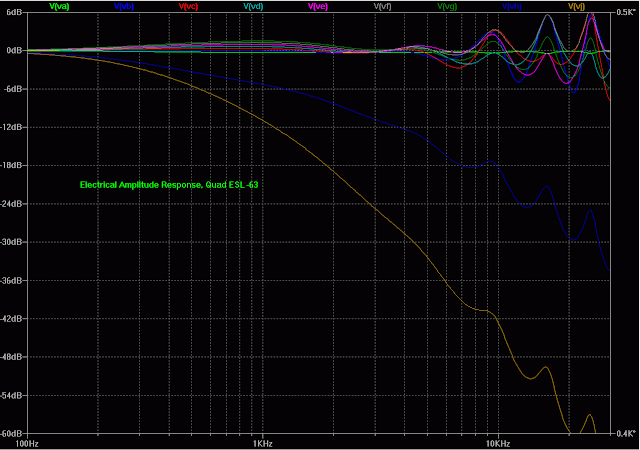Thursday, October 4, 2018

This was originally posted on my web site in 2006.  I'm in the process of transferring everything to this blog, so please forgive any weird formatting that results.

I've had a pair of Quad ESL-63s for about 6 years now and I've done all sorts of stuff to them including installing new diaphragms and rebuilding them into sturdier frames (made of PVC pipe, of course!).  Here's some miscellaneous photos, drawings, simulations, etc. I've either found elsewhere or done myself.

Simulations:

I used SwitcherCAD III (now called LTSpice) to simulate the function of the delay line in the speaker.  Here are some of the results:Simulation schematic of the Quad ESL-63.ESL-63 transient simulation at 50 Hz.ESL-63 transient simulation at 500 Hz.ESL-63 transient simulation at 5 kHz.  Sound travels at approximately 1000 m/s, so every us of delay represents about 1 mm physical offset.Frequency response, electrical, amplitude.Phase response, electrical.

The results are quite sensitive to the inductor coupling coefficient, but the value of the shorted inductance has almost no effect on the gross results.  At low frequencies the LC delay line is not very effective.  At higher frequencies it starts to work, but I was expecting longer delays.  Some additional research into the components used in the model is required.

Here is the spice netlist:

* C:\mark\ESL-63 Rebuild\ESL-63 simulation.asc
L1 VA VB {LD} Rser={LRser}
L2 VB VC {LD} Rser={LRser}
L3 VC VD {LD} Rser={LRser}
L4 VD VE {LD} Rser={LRser}
L5 VE VF {LD} Rser={LRser}
L6 VF VG {LD} Rser={LRser}
L7 N003 N009 {LD} Rser={LRser}
L8 N004 N003 {LD} Rser={LRser}
L9 N005 N004 {LD} Rser={LRser}
L10 N006 N005 {LD} Rser={LRser}
L11 N007 N006 {LD} Rser={LRser}
L12 N008 N007 {LD} Rser={LRser}
C1 VA N009 {Cseg}
C2 VB N003 {Cseg}
C3 VC N004 {Cseg}
C4 VD N005 {Cseg}
C5 VE N006 {Cseg}
C6 VF N007 {Cseg}
C7 VA N003 22p
C8 VB N009 22p
C9 VB N004 22p
C10 VC N003 22p
C11 VC N005 22p
C12 VD N004 22p
C13 VD N006 22p
C14 VE N005 22p
C15 VE N007 22p
C16 VF N006 22p
C17 VF N008 10p
C18 VG N007 10p
R1 VG N001 300k
R2 N002 N008 300k
C19 N001 N002 880p
R3 VG VH 360k
R4 VH VJ 360k
R5 N010 N008 360k
R6 N011 N010 360k
C20 VH N010 200p
C21 VJ N011 360p
V1 VA 0 SINE(0 100 {freq} 0 0 0 4) AC 1 Rser=3.1k
V2 0 N009 SINE(0 100 {freq} 0 0 0 4) AC 1 Rser=3.1k
L13 N012 N016 {LS} Rser=.05
R7 N012 N016 .05
R8 N016 0 10G
L14 N013 N017 {LS} Rser=.05
R9 N013 N017 .05
R10 N017 0 10G
L15 N014 N018 {LS} Rser=.05
R11 N014 N018 .05
R12 N018 0 10G
L16 N015 N019 {LS} Rser=.05
R13 N015 N019 .05
R14 N019 0 10G
L17 N020 N024 {LS} Rser=.05
R15 N020 N024 .05
R16 N024 0 10G
L18 N021 N025 {LS} Rser=.05
R17 N021 N025 .05
R18 N025 0 10G
L19 N022 N026 {LS} Rser=.05
R19 N022 N026 .05
R20 N026 0 10G
L20 N023 N027 {LS} Rser=.05
R21 N023 N027 .05
R22 N027 0 10G
L21 N028 N032 {LS} Rser=.05
R23 N028 N032 .05
R24 N032 0 10G
L22 N029 N033 {LS} Rser=.05
R25 N029 N033 .05
R26 N033 0 10G
L23 N030 N034 {LS} Rser=.05
R27 N030 N034 .05
R28 N034 0 10G
L24 N031 N035 {LS} Rser=.05
R29 N031 N035 .05
R30 N035 0 10G
;ac oct 50 100 30k
.param Cseg=11p
.param LD=3
K1 L1 L13 {COUP}
K2 L2 L14 {COUP}
K3 L3 L15 {COUP}
K4 L4 L16 {COUP}
K5 L5 L17 {COUP}
K6 L6 L18 {COUP}
K7 L7 L19 {COUP}
K8 L8 L20 {COUP}
K9 L9 L21 {COUP}
K10 L10 L22 {COUP}
K11 L11 L23 {COUP}
K12 L12 L24 {COUP}
.param  LS=.2
.param COUP=.5
.param LRser=200
.tran 0 {stopt} {startt}
* delay line inductor parameters
.param freq=3000
.param stopt=3/freq
.param startt=2/freq
* transient simulation parameters
* Mark Rehorst
* December 2006
* Speaker segment parameters
.backanno
.end